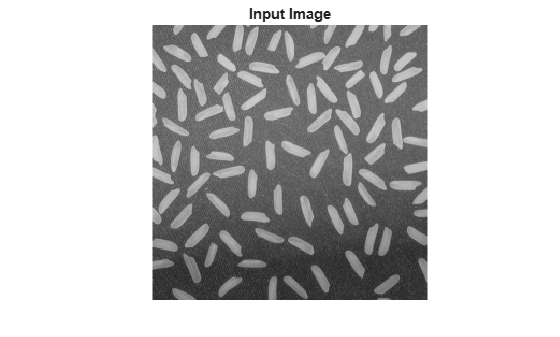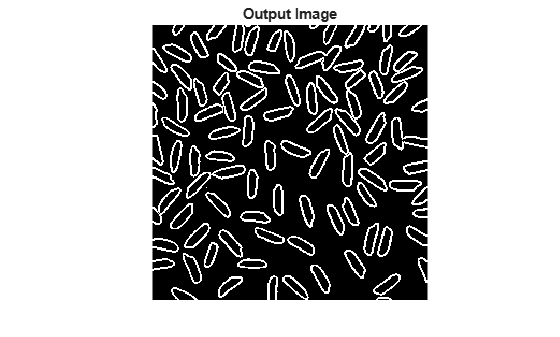# visionhdl.EdgeDetector

Find edges of objects

## Description

`visionhdl.EdgeDetector` finds the edges in a grayscale pixel stream using the Sobel, Prewitt, or Roberts method. The object convolves the input pixels with derivative approximation matrices to find the gradient of pixel magnitude along two orthogonal directions. It then compares the sum of the squares of the gradients to a configurable threshold to determine if the gradients represent an edge. The Sobel and Prewitt methods calculate the gradient in horizontal and vertical directions. The Roberts method calculates the gradients at 45 and 135 degrees.

The object returns a binary image, as a stream of pixel values. A pixel value of 1 indicates that the pixel is an edge. You can optionally enable output of the gradient values in the two orthogonal directions at each pixel.

Note

Starting in R2016b, instead of using the `step` method to perform the operation defined by the System object™, you can call the object with arguments, as if it were a function. For example, `y = step(obj,x)` and `y = obj(x)` perform equivalent operations.

## Construction

`ED = visionhdl.EdgeDetector` returns a System object, `ED`, that detects edges using the Sobel method.

`ED = visionhdl.EdgeDetector(Name,Value)` returns a System object, `ED`, with additional options specified by one or more `Name`,`Value` pair arguments. `Name` is a property name and `Value` is the corresponding value. `Name` must appear inside single quotes (`''`). You can specify several name-value pair arguments in any order as `Name1,Value1,...,NameN,ValueN`. Properties not specified retain their default values.

## Properties

 `Method` Edge detection algorithm. Specify `'Sobel'`, `'Prewitt'`, or `'Roberts'` method. Default: `'Sobel'` `BinaryImageOutputPort` Enable the `Edge` output of the `step` method. When this property is `true`, the `step` method returns a binary pixel value representing whether the object detected an edge at each location in the frame. Default: `true` `GradientComponentOutputPorts` Enable the `G1` and `G2` outputs of the `step` method. When this property is `true`, the `step` method returns two values representing the gradients calculated in two orthogonal directions at each pixel. Set the data type for this argument in the `GradientDataType` property. Default: `false` `ThresholdSource` Source for the gradient threshold value that indicates an edge. Set this property to `'Input port'`to set the threshold as an input argument to the `step` method. When this property is set to `'Property'`, set the threshold in the `Threshold` property. Default: `'Property'` `Threshold` Gradient threshold value that indicates an edge, specified as a numeric scalar value. The object compares the square of this to the sum of the squares of the gradients. The object casts this value to the data type of the gradients. This property applies when you set `ThresholdSource` to `'Property'`. Default: 20 `LineBufferSize` Specify a power of two that accommodates the number of active pixels in a single horizontal line. Choose a power of two that accommodates the number of active pixels in a horizontal line. If you specify a value that is not a power of two, the object uses the next largest power of two. The object allocates (N - 1)-by-`LineBufferSize` memory locations to store the pixels, where N is the number of lines in the differential approximation matrix. If you set the `Method` property to `'Sobel'`or `'Prewitt'`, then N is 3. If you set the `Method` property to `'Roberts'`, then N is 2. Default: 2048 `PaddingMethod` Select one of these methods for padding the boundary of the input image. For more information about these methods, see Edge Padding. `'Symmetric'` — Set the value of the padding pixels to mirror the edge of the image. This option prevents edges from being detected at the boundaries of the active frame.`'None'` — Exclude padding logic. The object does not set the pixels outside the image frame to any particular value. This option reduces the hardware resources used by the object and the blanking required between frames but affects the accuracy of the output pixels at the edges of the frame. To maintain pixel stream timing, the output frame is the same size as the input frame. However, to avoid using pixels calculated from undefined padding values, mask off the n/2 pixels around the edge of the frame for downstream operations, where n is the size of the operation kernel. For details, see Increase Throughput with Padding None. Default: `'Symmetric'` `RoundingMethod` Rounding mode used for fixed-point operations. The object uses fixed-point arithmetic for internal calculations when the input is any integer or fixed-point data type. This option does not apply when the input data type is `single` or `double`. Default: `Floor` `OverflowAction` Overflow action used for fixed-point operations. The object uses fixed-point arithmetic for internal calculations when the input is any integer or fixed-point data type. This option does not apply when the input data type is `single` or `double`. Default: `Wrap` `GradientDataType` Data type for the gradient output values, specified as `numerictype(signed,WL,FL)`, where WL is the word length and FL is the fraction length in bits. `'Full precision'` (default) — Use full-precision rules based on the data type of the `pixelIn` argument of the `step` method, and the coefficients of the derivative approximation matrices.`'custom'` — Use the data type defined in the`CustomGradientDataType` property. `CustomGradientDataType` Data type for the gradient output values, specified as `numerictype(signed,WL,FL)`, where WL is the word length and FL is the fraction length in bits. Default: `numerictype(1,8,0)`

## Methods

 step Detect edges at an image pixel
Common to All System Objects
`release`

Allow System object property value changes

## Examples

collapse all

Detect edges in a thumbnail image using the Sobel method.

Import a test image.

```frmInput = imread('rice.png'); [frmActivePixels,frmActiveLines] = size(frmInput); figure imshow(frmInput) title 'Input Image'```Create a serializer and specify the size of inactive pixel regions.

```frm2pix = visionhdl.FrameToPixels(... 'NumComponents',1,... 'VideoFormat','custom',... 'ActivePixelsPerLine',frmActivePixels,... 'ActiveVideoLines',frmActiveLines,... 'TotalPixelsPerLine',frmActivePixels+10,... 'TotalVideoLines',frmActiveLines+10,... 'StartingActiveLine',6,... 'FrontPorch',5);```

Create an edge detection object with the default property values. The default detection method is Sobel.

`edgeDetectSobel = visionhdl.EdgeDetector();`

Speed up simulation by enabling a simulation mode that uses code generation for the System object.

`edgeDetectSobel.simulateUsing('Code generation');`

Serialize the test image using the object you created. `pixIn` is a vector of intensity values. `ctrlIn` is a vector of control signal structures. Preallocate vectors for the output signals.

Note: This syntax runs only in R2016b or later. If you are using an earlier release, replace each call of an object with the equivalent `step` syntax. For example, replace `myObject(x)` with `step(myObject,x)`.

```[pixIn,ctrlIn] = frm2pix(frmInput); [~,~,numPixelsPerFrame] = getparamfromfrm2pix(frm2pix); ctrlOut = repmat(pixelcontrolstruct,numPixelsPerFrame,1); edgeOut = false(numPixelsPerFrame,1);```

For each pixel in the stream, compute whether it represents an edge.

```for p = 1:numPixelsPerFrame [edgeOut(p),ctrlOut(p)] = edgeDetectSobel(pixIn(p),ctrlIn(p)); end```

Create a deserializer with a format matching that of the serializer. Use the deserializer to convert the output pixel stream to an image frame.

```pix2frm = visionhdl.PixelsToFrame(... 'NumComponents',1,... 'VideoFormat','custom',... 'ActivePixelsPerLine',frmActivePixels,... 'ActiveVideoLines',frmActiveLines); [frmOutput,frmValid] = pix2frm(edgeOut,ctrlOut); if frmValid figure imshow(frmOutput) title 'Output Image' end```## Algorithms

This object implements the algorithms described on the Edge Detector block reference page.

| | (Image Processing Toolbox)

Introduced in R2015a

## SupportGet trial now